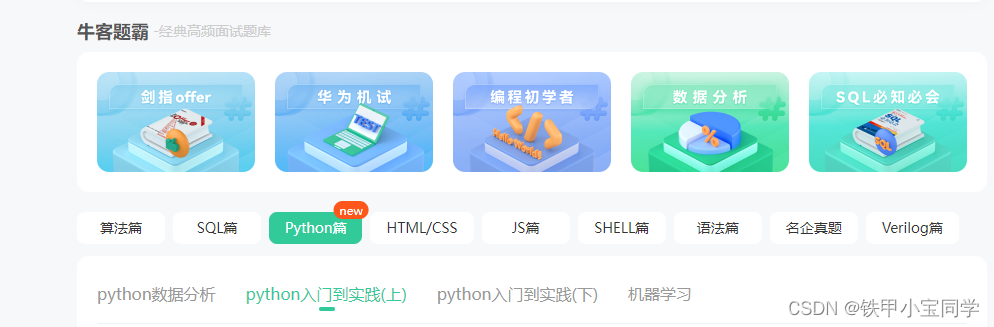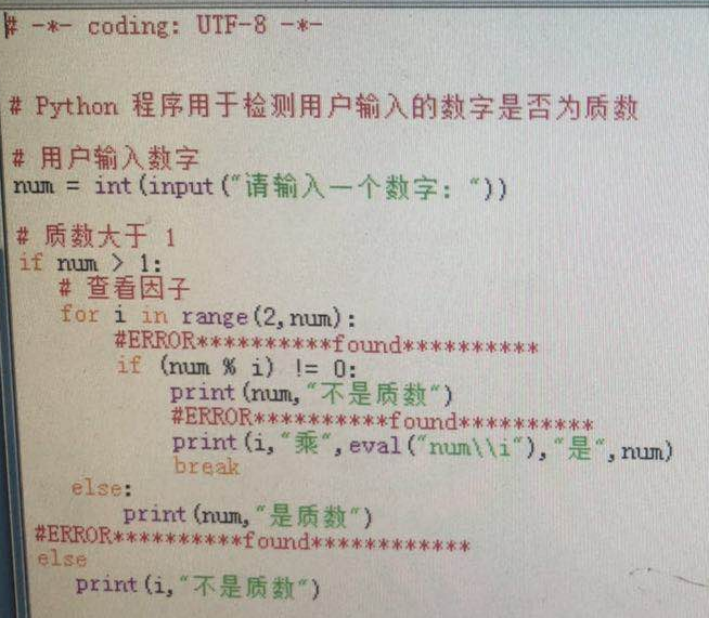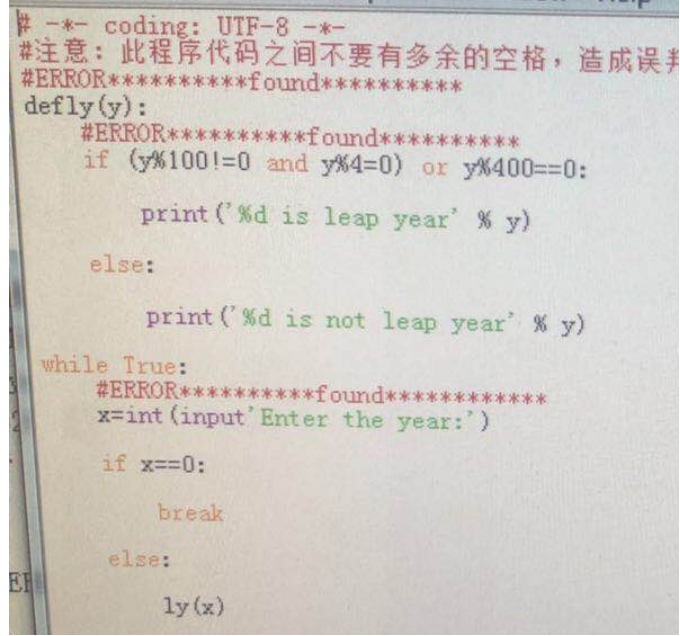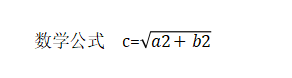### Python——基础习题（300题）

—— 致正在努力的你！

## 在线答题1：

2：

# 选择

## 单选题

1）：Python语言采用______来表明每行代码的层次关系。

A、注释和制表符

B、制表符或括号

C、括号

D、空格或制表符

2）：下列属于Python注释的方式为______。

A、–

B、//

C、#

D、/*…*/

3）：Python语言对于变量的命名要求严格，下面几个选项中非法的变量命名是______。

A、_var1

B、Var_1

C、$var_1 D、str1 答案：C 4）：下面不属于Python语言表示字符串的方式是______。 A、单引号 B、括号 C、三引号 D、双引号 答案：B 5）：下面变量正确的赋值方式是______。 A、x=1 B、int x;<回车>x=1 C、=x D、%x=1 答案：A 6）：我们应该了解到，python2到python3经历了很多重大改变，python3不属于接收用户输入的语句是______。 A、a=input(“input:”) B、input(“input”) C、a=”input:” D、b=input() 答案C 7）：下面程序运行后将输出______。 a=52 b=62 c=b+1 print(eval(“a+c”)) A、a+c B、b+1 C、115 D、”a+c” 答案C 8）：下面程序运行后输出的结果是______。 n=7 sum=0 for i in range(int(n)): sum+=i+1 print(“结果为：”,sum) A、结果为: 25 B、结果为: 28 C、结果为: 29 D、程序报错 答案B 9）：下面一段代码需要______保证其正常输出。 my_len(my_def): my_def.split() return len(my_def) res = my_len(‘hfweiie8832 fej中文’) print(res) A、首行缩进与第二行保持一致 B、首行添加def关键字，且用空格与函数名隔开 C、第三行去掉len关键字 D、无需修改，程序可以正常输出 答案：B 10）：下列明显不属于画笔控制函数的是______。 A、turtle.speed() B、turtle.forward() C、turtle.goto() D、turtle.screensize() 答案：D 11）：如果你想绘制五星红旗，你需要导入的包______。 A、turtle和pip B、wheel C、turtle和math D、NLTK和turtle 答案：C 12）：以下程序下运行后将输出______。 a=”””dsad dsafsda “”” print(a) A、dsaddsafsda B、dsad dsafsda C、””dsaddsafsda”” D、dsad\ndsafsda\n 答案B 13）：下列不属于python3中保留字的是______。 A、elseif B、del C、raise D、class 答案A 14）：变量名字的第一个字符必须是______。 A、数字或字母 B、数字或下划线 C、字母 D、字母或下划线 答案：D 15）：下面这段代码是一个死循环，如何让这个程序执行后不输出结果并永远处于运行状态______。 z=2 while z>1: z = z+1 print(z) A、首行缩进四个空格 B、末行取消缩进 C、第二行改为z<1 D、第三行z+=z+1 答案：B 1．下列哪个语句在Python中是非法的？（ B ） A、x = y = z = 1 B、x = (y = z + 1) C、x, y = y, x D、x += y 2．关于Python内存管理，下列说法错误的是（B ） A、变量不必事先声明 B、变量无须先创建和赋值而直接使用 C、变量无须指定类型 D、可以使用del释放资源 3、下面哪个不是Python合法的标识符（B ） A、int32 B、40XL C、self D、__name__ 4、下列哪种说法是错误的 （ A ） A、除字典类型外，所有标准对象均可以用于布尔测试 B、空字符串的布尔值是False C、空列表对象的布尔值是False D、值为0的任何数字对象的布尔值是False 5、Python不支持的数据类型有 （ A） A、char B、int C、float D、list 6、关于Python中的复数，下列说法错误的是（C ） A、表示复数的语法是real + image j B、实部和虚部都是浮点数 C、虚部必须后缀j，且必须是小写 D、方法conjugate返回复数的共轭复数 7、关于字符串下列说法错误的是 （ B） A、字符应该视为长度为1的字符串 B、字符串以\0标志字符串的结束 C、既可以用单引号，也可以用双引号创建字符串 D、在三引号字符串中可以包含换行回车等特殊字符 8、以下不能创建一个字典的语句是 （ C ） A、dict1 = {} B、dict2 = { 3 : 5 } C、dict3 = {[1,2,3]: “uestc”} D、dict4 = {(1,2,3): “uestc”} 9、下列Python语句正确的是 （ D ） A、min = x if x < y else y B、max = x > y ? x : y C、if (x > y) print x D、while True : pass 10 计算机中信息处理和信息储存用（A） A 二进制代码 B 十进制代码 C 十六进制代码 D ASCII代码 11 python源程序执行的方式（B） A 编译执行 B 解析执行 C 直接执行 D边编译边执行 12 Python语言语句块的标记是( C ) A 分号 B 逗号 C 缩进 D / 13 以下是字符转换成字节的方法是（B） A decode() B encode() C upper() D rstrip() “ab”+”c”*2 结果是：（C） A abc2 B abcabc C abcc D ababcc 14 以下会出现错误的是（B） A ‘北京’.encode() B ‘北京’.decode() C ‘北京’.encode().decode() D 以上都不会错误 15.如下： str1 = “Runoob example….wow!!!” str2 = “exam”; Print(str1.find(str2, 5)) 打印的结果是（B） A 6 B 7 C 8 D -1 调用以下函数返回的值(D) def myfun(): pass A 0 B 出错不能运行 C 空字符串 D None 16 函数如下： def showNnumber(numbers): for n in numbers: print(n) 下面那些在调用函数时会报错（ C） A showNumer([2,4,5]) B showNnumber(‘abcesf’) C showNnumber(3.4) D showNumber((12,4,5)) 17 函数如下 def chanageInt(number2): number2 = number2+1 print(“changeInt: number2= “,number2) #调用 number1 = 2 chanageInt(number1) print(“number:”,number1) 打印结果哪项是正确的（ B ） A changeInt: number2= 3 number: 3 B changeInt: number2= 3 number: 2 C number: 2 changeInt: number2= 2 D number: 2 changeInt: number2= 3 18.关于python类 说法错误的是（B） A 类的实例方法必须创建对象后才可以调用 B 类的实例方法必须创建对象前才可以调用 C 类的类方法可以用对象和类名来调用 D 类的静态属性可以用类名和对象来调用 19.定义类如下 class Hello(): def __init__(self,name) self.name=name def showInfo(self) print(self.name) 下面代码能正常执行的 ( C ) A h = Hello h.showInfo() B h = Hello() h.showInfo(‘张三’) C h = Hello(‘张三’) h.showInfo() D h = Hello(‘admin’) showInfo 20.以下哪项python能正常启动（D） A 拼写错误 B 错误表达式 C 缩进错误 D 手动抛出异常 21 对以下程序描述错误的是（A） try: #语句块1 except IndexError as i: # 语句块2 A 改程序对异常处理了，因此一定不会终止程序 B 改程序对异常处理了，不一定不会因异常引发终止 C 语句块1，如果抛出IndexError 异常，不会因为异常终止程序 D 语句块2 不一定会执行 22 程序如下： try: number = int(input(“请输入数字：”)) print(“number:”,number) print(“=======hello======”) except Exception as e: # 报错错误日志 print(“打印异常详情信息： “,e) else: print(“没有异常”) finally:#关闭资源 print(“finally”) print(“end”) 23.输入的是 1a 结果是：( B ) A number: 1 打印异常详情信息： invalid literal for int() with base 10: finally end B 打印异常详情信息： invalid literal for int() with base 10: finally end C ========hello=========== 打印异常详情信息： invalid literal for int() with base 10: finally End D 以上都正确 24 导入模块的方式错误的是（D） A import mo B from mo import * C import mo as m D import m from mo 25 以下关于模块说法错误的是（C） A 一个xx.py就是一个模块 B 任何一个普通的xx.py文件可以作为模块导入 C 模块文件的扩展名不一定是 .py D 运行时会从制定的目录搜索导入的模块，如果没有，会报错异常 1. S=’1234567890’,以下表示’1234’的选项是 A.s[1:5] B.s[0:4] C.s[-10:-5] D.s[0:3] 1. 以下代码的输出结果是 for i in range(1,6): if i%4 == 0: break else: print(i,end=”,”) A.1,2,3 B.1,2,3,5 C.1,2,3,5,6 D.1,2,3,4 1. 无限循环语句 While 5: 关于这条语句说法正确的是 A.while后只能是逻辑表达式 B.while 5:语句是一条正确的语句C.while后不能给常量D.while后只能是关系表达式 1. Print(100-25*3%4)应该输出 A.97 B.25 C.1 D.0 1. 在Python语言中，可以作为源文件后缀名的是 A.py B.png C.PPt D.pdf 1. 以下变量名，不符合Python语言变量命名规则的是 A.keyworld33_ B.keyworld_33 C.33_keyworld D._33keyworld 1. s, t=”love”, ”python” s+=t s[-1],s[::2],s[-2::-3] 正确的结果是 A.’n’,’vpto’,’opl’ B.’n’,’lvpto’,’oyv’ C.’n’,’lvpto’,’nte’ D.’n’,’vpto’,’oyv’ 1. 以下代码的输出结果是 for s in “HelloWorld”: if s == “W”: Continue print(s, end=””) A. HelloWorld B. Helloorld C. World D. Hello 1. 以下不是Python语言所使用特殊含义符号的是 A.* B.$          C.**       D.&

1. 在Python语言中，不能作为变量名的是

A.3p   B._fg   C. p  D. Temp

1. 3*9+2**5//(6/2)+8%3-5表示运算的结果是

A.33.0     B.-5        C.34.0       D.35.0

1. s=0

i=1

while 5:

s=s+i

i=i+1

If i==101:

break

print(s)程序段的功能是

A. 求100（含100）以内自然数的和

B. 求100（含100）以内各自然数的阶乘之和

C. 求100（含100）以内偶数的和

D. 求100（含100）以内奇数的和

1. 在Python语言中，IPO模式不包括

A.Input（输入） B.Process（处理） C.Output（输出） D.Program（程序）

1. 以下语句的执行结果是

y1=’’

y2=’ ‘

print(y1.isspace(),y2.isspace())

A. True False   B. False True  C. True True  D. False False

1. len(“hello world!”)的输出结果为

A.12 B.10 C.9 D.11

1. 以下不是Python语言保留字的是

A.false  B.as  C.def   D.from

1. 计算机有两个基本特性

A.数据处理和存储  B.功能性和可编程性C.自动性和准确性 D.输入和输出

1. 以下构成Python循环结构的方法中, 正确的是

A. if B. loop C. while D. do…for

1. 以下不是python的注释方式是

A. #注释第一行 #注释第二行 B. #注释第一行 C.”’python文档注释’” D.//注释第一行

20. 对负数取平方根，即使用函pow（-1,0.5),其x为负数,将产生

A.什么都不产 B. ValueError错误 C.虚数 D.程序崩溃

21. 关于异常处理机制,下列说法中最合理的是

A. 用if判断代替所有的try- except结构

B. 应当大量使用以避免任何可能的错误

C. 将所有代码放到一个try语句块中避免程序报错

D.结合函数设计统筹应用

22. 0xf2是——进制的数。

A.八进制 B.二进制 C.十进制 D.十六进制

23. 下面语句的执行结果是

tstr=’Baidu Translation is an online translation service’

print(len(tstr.split(‘a’)))

A.6 B.9 C.7 D.8

24. 以下代码的输出结果是print（0.1+0.2==0.3)

A.True B.0 C.-1 D.False

25. 以下关于Python循环结构的描述中，错误的是

A. Python通过for、while等保留字构建循环结构

B.break用来结束当前当次语句，但不跳出当前的循环体

C.遍历循环中的遍历结构可以是字符串、文件、组合数据类型和range（）函数等

D.continue只结束本次循环

26. 以下关于Python字符编码的描述中,错误的是

A. Python字符编码使用ASCII编码

B. print(ord(‘a’))输出97

C. chr(x)和ord (y)函数用于在单字符和Unicode编码值之间进行转换

D. print(chr(65))输出A

27. import mathmath. factorial（5)和math. gcd (36, 24)两个函数输出的结果

A.120，36 B.120，12 C.5，12 D.5，24

28. 关于表达式中整型、浮点型、复数型的运算结果类型说法正确的是

A. 表达式中整型,浮点型复数型运算结果是字符型

B. 表达式中整型、浮点型、复数型运算结果是整型

C. 表达式中型浮点型复数型运算结果是复数型

D. 表达式中整型、浮点型、复数型运算结果是浮点型

29.s=”Python”

Print(“{:*^10}”.format(s))的正确结果是

A.**Python B.**Python** C. Python D. Python**

30. 以下关于循环结构的描述,错误的是

A. 当try中有循环后构时,循环结构中出错，会跳出循环并进入except

B. continue语句执行时,会跳回continue所在的循环开头

C. for者whileelse配使用的时候，循环非正常结束时会执行else

D. break语句执行时，会跳出break所在的第一层循环

31. 以下选项不属于Python语言特点的是

A. 平台无关 B. 适合编写系统软件 C. 语法简洁 D. 第三方库丰富

32. 表达式eval(‘500//10’)的结果是

A.500/10 B.’500/10’ C.50.1 D.50

33. 下面代码的输出结果是s=” The python language is a cross platform language.”print (s. find (‘language’.30)

A.40 B.10 C.系统报错 D.11

34.try-except结构中，——会执行except对应的语句块

A.正常程序结束后B. try中语句有循环时C. try中语句出现异常时 D. try中语句有分支时

35.Python 3.x版本的保留字总数是

A.33 B.29 C.27 D.16

36.以下代码的输出结果是

A=10.99 print(complex(a))

A.0.99 B.10.99+j C.0.99+j D.(10.99+0j)

37.x**=3语句表示的是

A. x=3 B. x=x**3 C. x*3 D. x**3

38.以下关于分支结构的描述中，错误的是

A. if语句中条件部分可以使用任何能够产生True和False的语句和函数

B.多分支结构用于设置多个判断条件以及对应的多条执行路径

C.二分支结构有一种紧凑形式，使用保留字if和elif实现

D. if语句中语句块执行与否依赖于条件判断

39.”世界很大”+”人很渺小”的输出结果是

A. ”世界很大”+”人很渺小”B. 世界很大+人很渺小C. 世界很大人很渺小D. ”世界很大””人很渺小”

40. 遍历循环语句for i in range（n）关于n的数据类型说出正确的是

A.字符串型B.浮点型C.复数类型D.整数类型

41. 以下代码的输出S= ‘Pame’

for i in range(len(S)):

print(S[-i],end=””)

A. ameP B. Pame C. emaP D.Pema

42.以下不属于Python语言保留字的是

A. pass B. except C. True D. goto

43.程序如下

s=0

for i in range(1,101):

s=s+i

i=i+1

print(s)

A.100以内奇数的和

B.其它三项都不对

C.100以内偶数的和

D.100以内自然数的和

44.以下保留字不属于分支或循环逻辑的是

A. while B. in C. elif D. for

45.以下程序的输出结果是

x=4

ca=’123456’

if str(x) in ca:

print(ca.replace(ca[x],str(x-2)))

A.2 B.5 C.123426 D.123456

46.以下选项中描述正确的是

A.条件24<=28<25是不合法的

B.条件24<=28<25是合法的，且输出为True

C.条件24<=28<25是合法的，且输出为False

D.条件35<=45<75是合法的，且输出为False

47.以下——关于and的运算结果是True

A.(False and False == False B.(True and False)== True

C. False and True)== False  D.(True and True)== True

48. Python为源文件指定的默认字符编码是

A.GBK B. GB2312 C. UTF-8 D. ASCII

49.Python解释器在语法上不支持——编程方式

A.语句B.面向过程C.自然语言D.面向对象

50. 关于Python程序格式框架的描述,以下选项中错误的是

A. Python语言的缩进可以采用Tab键实现

B. 判断、循环、函数等语法形式能够通过缩进包含一批Python代码，进而表达对应的语义

C. Python单层缩进代码属于之前最邻近的一行非缩进代码,多层缩进代码根据缩进关系决定所属范围

D. Python语言不采用严格的“缩进“来表明程序的格式框架

51.Python语言通过——来体现语句之间的逻辑关系

A.缩进B.自动识别逻辑C.（）D.{ }

52. 以下描述错误的是

A. 编程语言中异常和错误是完全相同的概念

B. Python通过try、except等保留字提供异常处理功能

C. try-except可以在函数,循环体中使用

D. 当Python脚本程序发生了异常,如果不处理，运行结果不可预测

53.给出如下代码，可以输出“Python”的是

S=’Python is beautiful!’

A.print(s[-21:-14].lower) B.print(s[1:6]) C.print(s[:-14]) D.print(s[0:6].lower())

54. Python语言中用来表示代码块所属关系的语法是

A.缩进B.冒号C.花括号D.括号

55.eval（）函数的功能是

A.把整型转换为浮点型B.去掉括号内字符串的双引号“”或单引号‘’或三引号‘’‘ ’‘’C.把数字型转换为字符串型D.浮点型转为整型

56.以下哪个选项不是python语言的保留字

A. False B. and C. true D. if

57.整型和浮点型的区别

A.浮点型取值范围没有限制B.整型取值范围都限制C.浮点型和整型取值范围都限制D.整型取值范围没有限制

58.拟在屏幕上打印输出Hello World，使用python语句是

A. print（’Hello World’B. printf(‘Hello World’) C. print(Hello World) D. print(“Hello World”)

59.函数chr（x）的作用是

A.返回数字x作为Unicode编码对应的字符

B.返回数字x的十六进制字符串形式

C.返回字符x对应的Unicode值

D.返回字符串x中每个字符对应的Unicode编码值

60. ——是语句print(‘\nPython’)的运行结果

A.先输出n，然后新的一行输出Python

B.在新的一行输出：Python

C.直接输出：\nPython

D.直接输出：’\nPython’

61.程序如下

S=0

For I in range(1,11):

S=s+i

I=i+1

Print(s)

A. for I in range(1,11): B. s=s+iC. print(s) D. i=i+1

62.”世界那么大，我想去看看”[7：-3]输出

A.我想去B.我想C.想去D.

63.以下关于“for in“的描述，错误的是

A. for i in range(1,10,2)表示循环5次，i的值是从1到9的奇数

B. —用2,31和P*1.12311的时候,循环的次数是一样的

C. 使用range(a,B)函数指定for话环的循环变量取值是从a到b-1

D. 这个循环体语句中不能有break语句，会影响循环次数

64. 变量sstr =’天使的翅膀带着我在尘世飞翔’，执行下列选项,不能输出’翅膀’的是

A.print(sstr[-10:-8])B.print(sstr[3:-8])C.print(sstr[3:5])D. print(sstr[3::2])

65. 计算机CPU可以直接理解和执行——类型的程序设计语言。

A. 汇编语言 B.机器语言C.高级语言D.Python程序设计语言

66.s=”python”   s.find(“h”)正确的结果是

A.3 B. h C. t D.4

67. 以下关于Python字符串的描述中, 错误的是

A.字符串’my\\text.dat’中第一个\表示转义符

B.空字符串可以表示为“’或’“

C. Pytnon字符串中,可以混合使用正整数和负整数进行索引和切片

D. python字符串采用[N:M]格式进行切片，获取字符串从索引N到M的子字符串（包含N和M）

68.变量tstr=’kip520’,表达式eval(tstr)3:-1])的结果是

A.p52 B.52 C.520 D.p520

69.程序如下

S=0

For I in range(1,11)

S=s+i

Print(s,i)

A.55，10 B.50，12 C.55，11 D.50，10

70.关于python的数字类型，以下选项中描述错误的事

A.浮点数也有十进制、二进制、八进制和十六进制等表示方式B.1.0是浮点数，不是整数C.复数类型虚部为0时，表示为1+0jD.整数类型的数值一定不会出现小数点

71.python通过——来判断当前程序是否在分支结构中

A.缩进B.花括号C.括号D.冒号

72.以下程序的输出结果是

X=3.1415926

Print（round（x，2），round（x））

A.3.14,3 B.3,3.14 C.2,2 D.6.283

73. 关于Python循环结构,以下选项中描述错误的是

A. 遍历循环中的 遍历结构可以是字符串、文件、组合数据类型和range（）函数等

B. break用来跳出最内层for或者lwhile循环，脱离该循环后程序从循环代码后继续执行C. 每个continue语句只有能力出当前层次的循环

D. python通过for、while等保留字提供遍历循环和无限循环结构

74.表达式divmod（40，3）的结果是

A.13 B.13，1 C.1 D.（13，1）

75.以下选项不属于Pvthon语言特点的是

A. 网络编程语言 B. 跨平台 C. 开源理念 D.优异的扩展性

76. 关于表达式中整型、浮点型、复数型的运算结果类型说法正确的是

A. 表达式中整型、浮点型、复数型达算结果是字符型

B. 表达式中整型、浮点型复数型运算结果是整数

C. 表达式中整型、浮点型、复数型运算结果是复数型

D. 表达式中整型、浮点型、复数型运算结果是浮点型

77.执行下列选项，输出了’Hello World’的选项是

A. print(“’Hello World’”) B. print(‘”Hello World”’) C. print(‘\“Hello World\”’) D. print(‘Hello World\”)

78.以下——数字是八进制的。

A.Ob072 B.0o711 C.Ox456 D.Oa1010

79.以下变量名中，不符合python语言变量命名规则的是

A.33_keyword B.keyworld33_ C.keyworld_33 D._33keyworld

80. 设str1=’*@python@*’,语句print（str1[2:].strip(‘@’)）的执行结果是

A.*@python@* B.*python* C.python* D.python@*

81.以下不是python语言所使用特殊含义符号的是

A.? B.^ C.&= D.**

82. 关于表达式id(‘45′)的结果的描述, 错误的是

A.是一个正整数B. 可能是4539670688 C. 是’45’的内存地址 D.是一个字符串

83.表达式3*4**2//8%7的计算结果是

A.3 B.5 C.4 D.6

84.以下代码的输出结果是

While True:

Guess=eval(input())

If guess == 0x452//2:

Break

Print(guess)

A. break B.”0x452//2” C.0x452 D.553

85.以下代码的输出结果是

X=2+9*((3*12)-8)//10

Print(x)

A.27 B.28.2 C.27.2 D.26

86.若使以下程序执行结果是144，空格处该填写的代码是

x=’bee’

y=’ ’

for I in x:

y+=str(_____)

print(y)

A. ord(i)-ord(‘a’) B. ord(x)-ord(‘a’) C. i-’a’ D. chr(i)-chr(‘a’)

87. 关于Python语言,——说法是不正确的

A. Python语言由Guido van Rossum设计并领导开发

B. Python语言的使用不需要付费，不存在商业风险

C. Python语言由PSF组织所有，这是一个商业组织

D. Python语言提供开放开源理念

88.下面代码的输出结果是

Print（3>2）

A.0 B.1 C. False D. True

89.以下选项中，不是python语言保留字的是

A. pass B. do C. except D. while

90.关于python语言的描述，错误的选项是

A.是一种机器语言 B.支持面向对象 C.是一种解释类型的语言 D.支持面向过程

91.
weight=65

hight=1.75

print（”体重%d公斤，身高%.2f米。”%(weight,height)）运行后正确结果是

A.体重65%，身高1.75米。 B.体重65公斤，身高1.76米。 C.体重65公斤，身高1.7米。 D.体重65公斤，身高1.75米。

92.以下程序的输出结果是

for t in “Nation”:

for k in range(2):

if i==’n’:

break

print(i,end=””)

A. aattiioo B. NNaattiioo C. aattiioonn D.Naattiioon

93.python使用缩进作为语法边界，一般建议怎样缩进

A.2个空格 B.1个空格 C.8个空格D.4个空格

94.random库中用于生成随机小数的函数是

A. randrange() B. random() C. randint() D. getrandbits()

95. 以下关于Python语言中try语句的描述中，错误的是

A. 一个try代码块可以对应多个处理异常的except代码块B. try用来捕捉执行代码发生的异常，处理异常后能够回到异常处继续执行C. 当执行try代码块触发异常后，会执行except后面的语句D. try代码块不触发异常时，不会执行except后面的语句

96.假设x=2，x*=3+5**2的运算结果是

A.3 B.28 C.56 D.25

97.以下关于python语言浮点数类型的描述中，错误的是

A.浮点数类型表示带有小数的类型B. Python语言要求所有浮点数必须带有小数部分C.浮点数类型与数学中实数的概念一致D.小数部分不可以为0

98.——函数可以同时作用于数字类型和字符串类型

A. bin() B. len() C. complex() D. type()

99.score=eval(input(“请输入成绩：”))

if score>=60:

elif score>=70:

elif score>=80:

else score>=90:

A.良好 B.及格 C.中等 D.优秀

100.random库采用更多随机数生成算法是

A.线性同余法B.蒙特卡洛方法C.平方取中法D.梅森旋转算法

## 多选：

A: 一个元组是一个固定列表

B: 不能对元组中的元素进行添加、删除或替换

C: 由于元组是一个序列，所以序列的常用操作可以用于元组

D: 如果元组的所有元素都是不可变的，那么这个元组是不可变的

A: 类定义了对象的属性，并提供了用于初始化对象的初始化程序和操作这些属性的方法

B: 对象是类的一个实例

C: 初始化程序以__init__命名

D: 类的抽象是将类的实现和类的使用分离开来

A: 类的继承可以从现有的类派生出新类

B: 可以使用isinstance函数测试一个对象是否是一个类的实例

C: 多态意味着一个子类对象可以传递给一个需要父类类型的参数

D: 类之间常见的关系是关联、聚合、组合和继承

A: 无参数，无返回值

B: 无参数，有返回值

C: 有参数，无返回值

D: 有参数，有返回值

A: init:初始化函数

B: len:？获得长度

C: del:？析构函数，释放对象时使用

D: cmp:？比较运算

以下是正确的字符串（BD）

A  ‘abc”ab”   B ‘abc”ab’  C “abc”ab”  D “abc\”ab”

A count() 方法用于统计字符串里某个字符出现的次数

B  find() 方法检测字符串中是否包含子字符串 str  如果包含子字符串返回开始的索引值，否则会报一个异常

C  index() 方法检测字符串中是否包含子字符串 str， 如果str不在 返回-1

D 以上都错误

def chanageList(list):

list.append(” end”)

print(“list”,list)

#调用

strs =[‘1′,’2’]

chanageList(strs)

print(“strs”,strs)

A strs [‘1′,’2’]                 B list  [‘1′,’2’]

C list [‘1′,’2’,’end’]         D strs  [‘1′,’2’,’end’]

class  Hello():

pass

A 该类实例中包含__dir__（）方法

B 该类实例中包含__hash__（）方法

C 该类实例中只包含__dir__（），不包含__hash__（）

D 该类没有定义任何方法，所以该实例中没有包含任何方法

class hello():

def showInfo(sef):

print(self.x)

A该类不可以实例化

B 该类可以实例化

C 在pycharm 工具中会出现语法错误，说self没有定义

D 该类可以实例化，并且能正常通过对象调用showInfo()

# 填空

Python内置函数________用来返回序列中的最小元素

Python内置函数_____________用来打开或创建文件并返回文件对象。

Python内置函数_____________用来返回文件下一行.

Queue模块允许用户创建一个可以用于多个线程之间_________的队列数据结构

# 判断

1）：类名首字母一般采用大写，对象名采用小写。

2）：函数名通常采用小写，并用下划线或单词首字母大写增加名称可读性，导入的函数不以模块名做前缀。

3）：函数之间或类的方法之间用空行分割，表示一段新的代码的开始。

4）：程序中用于分隔各部分功能的空行也是代码的一部分。

5）：python3程序运行中会跳过注释行的内容，并且每个python文件前需要加上注释说明。

7）：k=”789″,b=”123″,eval(k+b)的值为921。

8）：a=5<回车>b=3<回车>c=a+b<回车>d=c+3。d的值输出为11。

9）：n=input(“请输入一个自然数：”)<回车>a=n+1<回车>print(a）可以正确输出a的值。

10）：is不是python的保留字。

11）：分支语句是控制程序运行的一类重要语句，它的作用是根据判断条件选择程序执行路径。

12）：print()函数和format()方法不可以将结果以特定格式输出。

13）：循环语句是控制程序运行的一类重要语句，与分支语句控制程序执行类似，它的作用是根据判断条件确定一段程序是否再执行一次或者多次。

14）：input(),print(),eval()是python的内置函数。

15）：函数是一组表达特定功能表达式的封装，只能够接受变量。

Python中的循环语句有 for , while和do…while

Python 中用 elif 代替了 else if，所以if语句的关键字为：if – elif – else

Python中for循环可以遍历任何序列的项目，如一个列表或者一个字符串

Python条件语句是通过一条或多条语句的执行结果（True或者False）来决定执行的代码块

Python不支持多继承

Python中，如果你的父类方法的功能不能满足你的需求，你可以在子类重写你父类的方法

Python支持使用字典的“键”作为下标来访问字典中的值

Python内置函数max()用来返回序列中的最小元素

Python的OS模块中，os.close()是用来打开文件描述符

Python中，内置的repr()函数会对给定的对象调用__repr__()特殊方法，并返回相应结果

# 解答题

1 请描述列表和元组之间的区别，以及分别在他们之间转型？

List  和tuple 区别

不同点：

1 定义的方式

list[]     tuple()

2 是否可变

list可变   tuple() 不可变

3 list中有 append()，insert() 函数，tuple中没有

相同点：

都是有序的集合（容器）

List转为tuple：

temp_list = [1,2,3,4,5]

tuple 转为list：

temp_tuple = (1,2,3)

2 定义函数的规则？

1函数代码块以 def 关键词开头，后接函数标识符名称和圆括号()。

2任何传入参数和自变量必须放在圆括号中间。圆括号之间可以用于定义参数。

3函数的第一行语句可以选择性地使用文档字符串—用于存放函数说明。

4函数内容以冒号起始，并且缩进。

5 return [表达式] 结束函数，选择性地返回一个值给调用方。不带表达式的return相当于返回 None。

3 __new__和__init__的区别？

__new__是一个静态方法,而__init__是一个实例方法.

__new__方法会返回一个创建的实例,而__init__什么都不返回.

5 去掉old_list = [1,1,1,3,4 ]  中的重复元素

new_list = list(set(old_list))

6  用两个元素之间有对应关系的list构造一个dict

names = [‘jianpx’, ‘yue’]

ages = [23, 40]

m = dict(zip(names,ages))

# 程序修改

## 1：

5 是质数

程序中位于每个//ERROR**********found**********下的语句行有错误，请加以更正，使它能得出正确的结果。

注意：只能修改每个//ERROR**********found************下的那一行，不要改动程序中的其他内容。【第一错误】

【第二错误】

【第三错误】

## 2：

Enter the year:2018

2018 is not leap year

Enter the year:2020

2020 is leap year

Enter the year:注意：只能修改每个//ERROR**********found************下的那一行，不要改动程序中的其他内容。

【第一错误】

【第二错误】

【第三错误】

Python3中input需要加括号，input(‘Enter the year:’)

# 案例

## 程序1：数字组合

【程序描述】

【程序分析】

【程序实现】

for i in range(1,5):    		for j in range(1,5):        		for k in range(1,5):            		if i!=j and j!=k and i!=k:                		print(i,j,k)

【程序结果】

1 2 3

1 2 4

1 3 2

1 3 4

1 4 2

1 4 3

2 1 3

2 1 4

2 3 1

2 3 4

2 4 1

2 4 3

3 1 2

3 1 4

3 2 1

3 2 4

3 4 1

3 4 2

4 1 2

4 1 3

4 2 1

4 2 3

4 3 1

4 3 2

## 程序2：猜猜这个数是多少？

【程序描述】

【程序分析】

xx =（i+100）

yy=（i+268）

【程序实现】

import mathfor i in range(1,10000):    		x=int(math.sqrt(i+100))    		y=int(math.sqrt(i+268))    		if (x*x==i+100)and(y*y==i+268):        		print(i)

【程序结果】

21

261

1581

## 程序3：判断这是一年中的第几天？

【程序描述】

【程序分析】

【程序实现】

    year=int(input("请输入年份"))	month=int(input("请输入月份"))	day=int(input("请输入日期"))	months=(0,31,59,90,120,151,181,212,243,273,304,334)	if 0<month<=12:   	 	sum=months[month-1]	else:    		print('输入的月份有误')	sum+=day	leap=0	if (year%400==0) or ((year%4==0) and (year%100!=0)):    		leap=1    		if(leap==1)and(month>2):        		sum+=1	print("今天是第%d天"%sum)

【程序结果】

## 程序4：判断整数大小

【程序描述】

【程序分析】

【程序实现】

	x=int(input("请输入进行比较的第1个数："))	y=int(input("请输入进行比较的第2个数："))	z=int(input("请输入进行比较的第3个数："))    list=[x,y,z]	list.sort()	for i in range(len(list)):    		print(list[i])

【程序结果】

23

34

55

## 程序5：斐波那契数列

【程序描述】

【程序分析】

F0 = 0     (n=0)

F1 = 1    (n=1)

Fn = F[n-1]+ F[n-2](n=>2)

【程序实现】

 def fib(n):     if n==1 or n==2:        return 1     return fib(n-1)+fib(n-2) print(fib(10))

【程序结果】

55

## 程序6：水仙花数

【程序描述】

【程序分析】

【程序实现】

for i in range(100,1000):     a=int(i/100)     b=int(i/10)%10     c=i%10     if i==a**3+b**3+c**3:         print(i)

【程序结果】

153

370

371

407

## 程序7：数字求和

【程序描述】

【程序分析】

1. 提示用户输入第1个数字
2. 提示用户输入第2个数字
3. 对两个数字进行相加
4. 输出两个数字相加的和

【程序实现】

num1=input("请输入第1个数字：")  num2=input("请输入第2个数字：")  sum=float(num1)+float(num2)  print("数字%s和%s相加之和是%.1f"%(num1,num2,sum))

【程序结果】

## 程序8：平方根

【程序描述】

【程序分析】

1. 提示用户输入一个数(必须将输入的数转为数字类型）
2. 使用math函数的sqrt计算这个数的平方根
3. 输出运算结果

【程序实现】

import math  num=int(input("请输入要计算平方根的数字："))  result=math.sqrt(num)  print("数字%d的平方根是%f"%(num,result))

【程序结果】

## 程序9：if语句

【程序描述】

【程序分析】

【程序实现】

num = float(input("输入一个数字: "))  if num > 0:     print("正数")  elif num == 0:     print("零")  else:     print("负数")

【程序结果】

## 程序10：阿姆斯特朗数

【程序描述】

1000以内的阿姆斯特朗数： 1, 2, 3, 4, 5, 6, 7, 8, 9, 153, 370, 371, 407。

【程序分析】

【程序实现】

# 获取用户输入的数字 num = int(input("请输入一个数字: ")) # 初始化变量 sum sum = 0 # 指数 n = len(str(num)) # 检测 temp = num while temp > 0:    digit = temp % 10    sum += digit ** n    temp //= 10 # 输出结果 if num == sum:    print(num,"是阿姆斯特朗数") else:    print(num,"不是阿姆斯特朗数")

【程序结果】

12 不是阿姆斯特朗数

153 是阿姆斯特朗数

## 程序11：输出指定范围的素数（else语句）

【程序描述】

【程序分析】

1. 提示用户输入指定的范围，分别是范围的最小值和最大值
2. 使用for循环在指定范围内进行循环
3. 由于满足素数的条件是只能被1和本身整除，所以可以使用if语句判断是否能整除，如果能，则输出素数。

【程序实现】

lower = int(input("输入区间最小值: ")) upper = int(input("输入区间最大值: ")) for num in range(lower,upper + 1): # 素数大于 1 if num > 1: for i in range(2,num): if (num % i) == 0: break else: print(num)

【程序结果】

2

3

5

7

11

13

17

19

23

29

31

37

41

43

47

## 程序12：生成日历

【程序描述】

【程序分析】

Calendar模块有很广泛的方法用来处理年历和月历，例如打印某月的月历。

【程序实现】

# 引入日历模块  import calendar  # 输入指定年月  yy = int(input("输入年份: "))  mm = int(input("输入月份: "))  # 显示日历  print(calendar.month(yy,mm))

【程序结果】

March 2017

Mo Tu We Th Fr Sa Su

1  2  3  4  5

6  7  8  9 10 11 12

13 14 15 16 17 18 19

20 21 22 23 24 25 26

27 28 29 30 31

## 程序13：统计字符串中的字符

【程序描述】

【程序分析】

1. 提示用户输入字符串
2. 声明四个变量，分别用于统计英文字母、空格、数字和其他字符的个数
3. 使用for循环遍历字符串中的字符，使用if判断语句判断遍历的字符满足哪个条件，如果满足条件，则对应的变量加1

【程序实现】

  str=input("请输入一个字符串:")  letters = 0  space = 0  digit = 0  others = 0  for c in str:      if c.isalpha():          letters += 1      elif c.isspace():          space += 1      elif c.isdigit():          digit += 1      else:         others += 1  print("字符串共有%d个英文字母，%d个空格，%d个数字，%d个其他字符               "%(letters,space,digit,others))

【程序结果】

## 程序14：文件的读取

【程序描述】

【程序分析】

1. 提示用户输入一个字符串
2. 使用字符串的upper方法将字符串的小写字母全部转换为大写字母
3. 使用write方法将字符串写入文件，需要注意的是，在写文件之前，首先要打开文件，完成写数据的操作后，要切记关闭文件。

【程序实现】

 fp = open('test.txt','w')  string = input('please input a string:\n')  string = string.upper()  fp.write(string)  fp = open('test.txt','r')  print(fp.read())  fp.close()

【程序输出】

Nice to meet you!

NICE TO MEET YOU!

## 程序15：十进制转二进制、八进制、十六进制

【程序描述】

【程序分析】

1. bin()：将十进制的数转为二进制
2. oct()：将十进制的数转为八进制
3. hex(): 将十进制的数转为十六进制

【程序实现】

# 获取用户输入十进制数 dec = int(input("输入数字：")) print("十进制数为：", dec) print("转换为二进制为：", bin(dec)) print("转换为八进制为：", oct(dec)) print("转换为十六进制为：", hex(dec))

【程序结果】

## 程序16： 生成10个两位的随机素数

【程序描述】

【程序分析】

Python的random模块提供了随机数生成方法random.randint(a,b),它返回一个大于等于a，小于等于b的随机整数。

【程序实现】

import  randomn=0while n<10:      x=random.randint(10,99)  #获得一个两位的随机整数      #判断x是否为素数      a=2      while a<x-1:          if x%a==0:         #若余数为0，说明x不是素数，结束当前循环               break            a+=1      else:          print(x)            #若正常结束循环时，说明x是素数，输出           n+=1                 #累计素数个数

【程序结果】（每次结果不一样）

61

29

83

41

73

97

19

11

31

17

## 程序17： 计算三角形面积

【程序描述】

【程序分析】

【程序实现】

  a = float(input('输入三角形第一边长: '))  b = float(input('输入三角形第二边长: '))  c = float(input('输入三角形第三边长: '))  # 计算半周长  s = (a + b + c) / 2  # 计算面积  area = (s*(s-a)*(s-b)*(s-c)) ** 0.5  print('三角形面积为 %0.2f' %area)

【程序结果】

## 程序18：字符串大小写转换

【程序描述】

【程序分析】

1. upper():将所有字符中的小写字母转换为大写字母
2. lower()：把所有字符中的大写字母转为小写字母
3. capitalize()：把第一个字母转化为大写字母，其余小写
4. title()：把每个单词的第一个字母转化为大写，其余小写

【程序实现】

  str = "www.runoob.com"  print(str.upper())          # 把所有字符中的小写字母转换成大写字母  print(str.lower())          # 把所有字符中的大写字母转换成小写字母  print(str.capitalize())     # 把第一个字母转化为大写字母，其余小写  print(str.title())          # 把每个单词的第一个字母转化为大写，其余小写

【程序结果】

WWW.RUNOOB.COM

www.runoob.com

Www.runoob.com

Www.Runoob.Com

## 程序19： 获取昨天日期

【程序描述】

【程序分析】

【程序实现】

# 引入 datetime 模块  import datetime  def getYesterday():     today=datetime.date.today()     oneday=datetime.timedelta(days=1)     yesterday=today-oneday     return yesterday  # 输出  print(getYesterday())

【程序结果】

2017-03-02

## 程序20： 变量交换

【程序描述】

【程序分析】

x,y = y,x

【程序实现】

# 用户输入 x = input('输入 x 值: ') y = input('输入 y 值: ') # 不使用临时变量 x,y = y,x print('交换后 x 的值为: {}'.format(x)) print('交换后 y 的值为: {}'.format(y))

【程序结果】

## 程序21： 质数判断

【程序描述】

【程序实现】

  # 用户输入数字  num = int(input("请输入一个数字: "))  # 质数大于 1  if num > 1:      # 查看因子      for i in range(2, num):          if (num % i) == 0:              print(num, "不是质数")              print(i, "乘于", num // i, "是", num)               break      else:          print(num, "是质数")  # 如果输入的数字小于或等于 1，不是质数  else:      print(num, "不是质数")

【程序结果】

12 不是质数

2 乘于 6 是 12

## 程序22： 简单计算器

【程序描述】

【程序分析】

1. 打印菜单，提示用户选择要进行的运算
2. 用户选择运算类型
3. 接收用户输入的值，用于参与运算
4. 输出运算的结果

【程序实现】

# 定义函数 def add(x, y):    """相加"""    return x + y def subtract(x, y):    """相减"""    return x - y def multiply(x, y):    """相乘"""    return x * y def divide(x, y):    """相除"""    return x / y # 用户输入 print("选择运算：") print("1、相加") print("2、相减") print("3、相乘") print("4、相除") choice = input("输入你的选择(1/2/3/4):") num1 = int(input("输入第一个数字: ")) num2 = int(input("输入第二个数字: ")) if choice == '1':    print(num1,"+",num2,"=", add(num1,num2)) elif choice == '2':    print(num1,"-",num2,"=", subtract(num1,num2)) elif choice == '3':    print(num1,"*",num2,"=", multiply(num1,num2)) elif choice == '4':    print(num1,"/",num2,"=", divide(num1,num2)) else:    print("非法输入")

【程序结果】

1、相加

2、相减

3、相乘

4、相除

2 + 3 = 5

1、相加

2、相减

3、相乘

4、相除

5 – 1 = 4

1、相加

2、相减

3、相乘

4、相除

2 * 5 = 10

1、相加

2、相减

3、相乘

4、相除

10 / 2 = 5.0

## 程序23： 最大公约数

【程序描述】

【程序实现】

# 定义一个函数  def hcf(x, y):         """该函数返回两个数的最大公约数"""     # 获取最小值    if x > y:         smaller = y     else:        smaller = x    for i in range(1,smaller + 1):        if((x % i == 0) and (y % i == 0)):            hcf = i    return hcf  # 用户输入两个数字  num1 = int(input("输入第一个数字: "))  num2 = int(input("输入第二个数字: "))  print( num1,"和", num2,"的最大公约数为", hcf(num1, num2))

【程序结果】

12 和 3 的最大公约数为 3

## 程序24： 最小公倍数

【程序描述】

【程序实现】

# 定义函数 def lcm(x, y):    #  获取最大的数    if x > y:        greater = x    else:        greater = y    while(True):        if((greater % x == 0) and (greater % y == 0)):            lcm = greater            break        greater += 1    return lcm # 获取用户输入 num1 = int(input("输入第一个数字: ")) num2 = int(input("输入第二个数字: "))   print( num1,"和", num2,"的最小公倍数为", lcm(num1, num2))

【程序结果】

12 和 2 的最小公倍数为 12

## 程序25： 字符串判断

【程序描述】

【程序分析】

1. isalnum(): 判断所有字符都是数字或者字母
2. isalpha(): 判断所有字符都是字母
3. isdigit():判断所有字符都是数字
4. islower():判断所有字符都是小写
5. isupper():判断所有字符都是大写
6. istitle():判断所有单词都是首字母大写，像标题
7. isspace():判断所有字符都是空白字符、\t、\n、\r

【程序实现】

print("测试实例一")  str = "www.itheima.com"  print(str.isalnum()) # 判断所有字符都是数字或者字母  print(str.isalpha()) # 判断所有字符都是字母  print(str.isdigit()) # 判断所有字符都是数字  print(str.islower()) # 判断所有字符都是小写  print(str.isupper()) # 判断所有字符都是大写  print(str.istitle()) # 判断所有单词都是首字母大写，像标题  print(str.isspace()) # 判断所有字符都是空白字符、\t、\n、\r  print("------------------------")  # 测试实例二  print("测试实例二")  str = "itheima"  print(str.isalnum())  print(str.isalpha())  print(str.isdigit())  print(str.islower())  print(str.isupper())  print(str.istitle())  print(str.isspace())

【程序结果】

False

False

False

True

False

False

False

————————

True

True

False

True

False

False

False

## 程序26： 合并文件数据

【程序描述】

【程序实现】

fp = open('test1.txt')  a = fp.read()  fp.close() fp = open('test2.txt') b = fp.read() fp.close() fp = open('test3.txt', 'w') l = list(a + b) l.sort() s = '' s = s.join(l) fp.write(s) fp.close()

【程序结果】

## 程序27： 猜数游戏

【程序描述】

【程序实现】

  import timeimport randomplay_it = input('do you want to play it.(\'y\' or \'n\')')while play_it == 'y':    c = input('input a character:\n')    i = random.randint(0, 2 ** 32) % 100    print('please input number you guess:\n')    start = time.clock()    a = time.time()    guess = int(input('input your guess:\n'))    while guess != i:        if guess > i:            print('please input a little smaller')            guess = int(input('input your guess:\n'))        else:            print('please input a little bigger')            guess = int(input('input your guess:\n'))    end = time.clock()    b = time.time()    var = (end - start) / 18.2    print(var)    if var < 15:        print('you are very clever!')    elif var < 25:        print('you are normal!')    else:        print('you are stupid!')    print('Congradulations')    print('The number you guess is %d' % i)    play_it = input('do you want to play it.')

【程序结果】

do you want to play it.(‘y’ or ‘n’)y

input a character:

5

60

……

29

28

3.81868131868e-05

you are very clever!

The number you guess is 28

do you want to play it.

## 程序28：为数据加密

【程序描述】

【程序实现】

from sys import stdouta = int(input('input a number:\n'))aa = []aa.append(a % 10)aa.append(a % 100 / 10)aa.append(a % 1000 / 100)aa.append(a / 1000)for i in range(4):    aa[i] += 5    aa[i] %= 10for i in range(2):    aa[i],aa[3 - i] = aa[3 - i],aa[i]for i in range(3,-1,-1):    stdout.write(str(aa[i]))

【程序结果】

input a number:

12

76.25.125.012

## 程序29：平方运算

【程序描述】

编写程序，求输入数字的平方，如果平方运算后小于 50 则退出。

【程序实现】

TRUE = 1FALSE = 0def SQ(x):    return x * xprint('如果输入的数字小于 50，程序将停止运行。')again = 1while again:    num = int(input('Please input number'))    print('运算结果为 %d' % (SQ(num)))    num=SQ(num)    if num >= 50:        again = TRUE    else:        again = FALSE

【程序结果】

## 程序30： 计算0-7组成的奇数个数

【程序描述】

【程序实现】

sum = 4s = 4for j in range(2,9):    print(sum)    if j <= 2:        s *= 7    else:        s *= 8    sum += sprint('sum = %d' % sum)

【程序结果】

4

32

256

2048

16384

131072

1048576

sum = 8388608

## 程序31：求值

【程序描述】

809*??=800*??+9*??+1 其中??代表的两位数,8*??的结果为两位数，9*??的结果为3位数。求??代表的两位数，及809*??后的结果。

【程序实现】

a = 809for i in range(10,100):    b = i * a + 1    if b >= 1000 and b <= 10000 and 8 * i < 100 and 9 * i >= 100:        print(b,'/',i,' = 809 * ',i,' + ', b % i)

【程序结果】

9709 / 12  = 809 *  12  +  1

## 程序32：猴子分桃

【程序描述】

【程序实现】

i = 0j = 1x = 0while (i < 5):    x = 4 * j    for i in range(0, 5):        if (x % 4 != 0):            break        else:            i += 1        x = (x / 4) * 5 + 1    j += 1print(x)

【程序结果】

3121.0

## 程序33：淘汰游戏

【程序描述】

【程序实现】

  nmax = 50n = int(input('请输入总人数:'))num = []for i in range(n):    num.append(i + 1)i = 0k = 0m = 0while m < n - 1:    if num[i] != 0 : k += 1    if k == 3:        num[i] = 0        k = 0        m += 1    i += 1    if i == n : i = 0i = 0while num[i] == 0: i += 1print(num[i])

【程序结果】

8

## 程序34：分解质因子

【程序描述】

【程序实现】

n=int(input('please input n:'))  result=[]  i=2  str1=str(n)+'='  while n>1:     if n%i==0:         n/=i         result.append(str(i))         i -= 1     i +=1  str1+='*'.join(result)  print(str1)  

【程序结果】

16=2*2*2*2

## 程序35：取整数右端的4~7位

【程序描述】

【程序分析】

(1)先使a右移4位。

(2)设置一个低4位全为1,其余全为0的数。可用~(~0<<4)

(3)将上面二者进行&运算。

【程序实现】

  a = int(input('input a number:\n'))b = a >> 4c = ~(~0 << 4)d = b & cprint('%o\t%o' % (a, d))

【程序结果】

input a number:

9

11 0

## 程序36：判断4位回文数

【程序描述】

【程序分析】

一个四位数abcd可以写为I1=a*1000+b*100+c*10+d

1. 用户输入一个整数I1
2. 分离千位、百位、十位、个位
3. 组合出新数字I2=d*1000+c*100+b*10+a
4. 比较I1==I2
5. 输出结果

【程序实现】

  I1=int(input("请输入一个四位数："))a=int(I1/1000)b=int(I1/100%10)c=int(I1/10%10)d=int(I1%10)I2=d*1000+c*100+b*10+aif I1==I2:    print("True")else:    print("False")

【程序结果】

True

False

## 程序37：汉诺塔（递归函数）

【程序描述】

【程序分析】

1. 如果只有一个盘子，则不需要利用B座，直接将盘子从A移动到C
2. 如果有2个盘子，可以先将盘2移动到B，将盘1移动到C后，再将盘2移动到C
3. 如果有3 个盘子，那么根据2个盘子的结论，可以借助C将盘2和盘3从A移动到B，将盘1从A移动到C，A变成空座；借助A座，将B上的两个盘子移动到C

将一个盘子从A移动到C

1. 盘子的数量从n变化到n-1，问题规模缩小了，显然n是一个可变的参数
2. 盘子的起始位置是变化的，等式左侧是A，右侧是A或B
3. 盘子的最终位置是变化的，等式左侧是C、右侧是B或C
4. 同样被借助的位置也是变化的

【程序实现】

def Hanoi(n,ch1,ch2,ch3):    if n==1:        print(ch1,'->',ch3)    else:        Hanoi(n-1,ch1,ch3,ch2)        print(ch1,'->',ch3)        Hanoi(n-1,ch2,ch1,ch3)N=int(input("请输入盘子的数量："))Hanoi(N,'A','B','C')

【程序结果】

A -> B

A -> C

B -> C

A -> B

C -> A

C -> B

A -> B

A -> C

B -> C

B -> A

C -> A

B -> C

A -> B

A -> C

B -> C

## 程序38：判断闰年

【程序描述】

【程序分析】

1. 能被4整除且不能被100整除的为闰年
2. 能被400整除的是闰年

【程序实现】

【程序结果】

## 程序39：寻找自幂数（38）

【程序描述】

【程序分析】

【程序实现】

#自幂数start=0end=0digit=0m=0n=int(input('请选择自幂数的位数【1，2，3，4，5，6】:'))while 0<n<7:    start=pow(10,n-1)    end=pow(10,n)-1    print(n,'位数的自幂数有:')    for k in range(start,end+1):        m=k        total=0        while m!=0:            digit=m%10            total+=pow(digit,n)            m=m//10        if total==k:            print(str(k),end=' ')    n=int(input('\n 请选择自幂数的位数【1，2，3，4，5，6】:'))else:    print('输入位数不在范围内,程序结束。')

【程序结果】

1 位数的自幂数有:

1 2 3 4 5 6 7 8 9

请选择自幂数的位数【1，2，3，4，5，6】:2

2 位数的自幂数有:

请选择自幂数的位数【1，2，3，4，5，6】:3

3 位数的自幂数有:

153 370 371 407

请选择自幂数的位数【1，2，3，4，5，6】:4

4 位数的自幂数有:

1634 8208 9474

请选择自幂数的位数【1，2，3，4，5，6】:5

5 位数的自幂数有:

54748 92727 93084

请选择自幂数的位数【1，2，3，4，5，6】:6

6 位数的自幂数有:

548834

请选择自幂数的位数【1，2，3，4，5，6】:

## 程序40：多维数据

【程序描述】

M=[[1,2,3],[‘a’,’b’,’c’],[7,8,9]]

1. 列表的每个维度长度可以不同
2. 列表的元素数据类型可以不同

123

456

789

【程序分析】

【程序实现】

a=[]for i in range(2):    a.append([])    for j in  range(2):        v=int(input("请输入元素："))        a[i].append(v)print(a)

【程序结果】

[[1, 2], [2, 3]]

## 程序41： 验证哥德巴赫猜想

【程序描述】

【程序分析】

【程序实现】

def main():    # 输入待验证的偶数    N=int(input("请输入待验证的偶数："))    while N<3 or N%2==1:        print("输入的数不符合要求")        N=int(input("请输入待验证的偶数n（n>2）："))    #生成素数表    Prime=set()    for i in range(2,N+1):        Prime.add(i)    for i in range(2,N+1):        if i in Prime:            for k in range(2*i,N+1,i):                if k in Prime:                    Prime.remove(k)    #验证该偶数能否分解为两个素数之和    for e in Prime:        f=N-e        if f>=e and f in Prime:            print(N,'=',e,'+',f)main()

【程序结果】

12 = 5 + 7

## 程序42： 解方程

【程序描述】

【程序分析】

【程序实现】

from math import *print("本程序求 ax^2+bx+c=0 的根")a=float( input("请输入 a:") )b=float( input("请输入 b:") )c=float( input("请输入 c:") )delta=b*b-4*a*cif(delta>=0):    delta=sqrt(delta)    x1=(-b+delta)/2/a    x2=(-b-delta)/(2*a)    print("两个实根分别为:",x1,x2)else:    print("没有实根")

【程序结果】

## 程序43： 异常处理

【程序描述】

【程序分析】

【程序实现】

k=0while(k<3):    try:        x=int(input('请输入第一个整数:'))        y=int(input('请输入第二个整数:'))        print('x/y=',x/y)    except ValueError:        print('请输入一个整数。')    except ZeroDivisionError:        print('除数不能为零。')    k=k+1

【程序结果】

x/y= 0.5217391304347826

## 程序44： 统计不及格人数（continue语句）

【程序描述】

【程序分析】

【程序实现】

x=[98,72,80,45,30,89,92,54,48,82,67,76]sum=0k=0;for item in x:    if(item<60):        continue    sum=sum+item    k=k+1;if(k!=0):    print("及格人数",k,"人,平均成绩是",sum/k)

【程序结果】

## 程序45： 求直角三角形的斜边

【程序描述】【程序分析】

【程序实现】

 from math import *a=float(input("请输入斜边 1 的长度")) #输入实数b=float(input("请输入斜边 2 的长度")) #输入实数c=a*a+b*b  #计算,得到的是斜边的平方c=sqrt(c) #开方，得到的是斜边长print("斜边长为:",c) #显示，一项是字符串，一项是 c 表示的斜边长

【程序结果】

## 程序46： 问年龄

【程序描述】

【程序分析】

【程序实现】

def fun(i): if i==1: return 10 return fun(i-1)+2 print(fun(5))

【程序结果】

18

## 程序47：求相同数字的和

【程序描述】

【程序分析】

【程序实现】

def test():    basis = int(input("输入一个基本的数字:"))    n = int(input("输入数字的长度:"))    b = basis    sum = 0    for i in range(0, n):        if i == n - 1:            print("%d " % (basis))        else:            print("%d +" % (basis))        sum += basis        basis = basis * 10 + b    print('= %d' % (sum))test()

【程序结果】

3 +

33 +

333 +

3333

= 3702

## 程序48：找出1000以内的所有完数

【程序描述】

【程序分析】

【程序实现】

from math import sqrtn = int(input('输入一个数字:'))sum = n*-1k = int(sqrt(n))for i in range(1,k+1):    if n%i == 0:        sum += n/i        sum += iif sum == n:    print('YES')else:    print('NO')

【程序结果】

YES

## 程序49：公民类

【程序描述】

【程序分析】

【程序实现】

class Citizen:    def __init__(self,id,name,age,sex):        self.id = id        self.name = name        self.age = age        self.sex = sexclass Student(Citizen):    def __init__(self,id,name,age,sex,stdno,grade,score):        super(Student, self).__init__(id,name,age,sex)        self.stdno = stdno        self.grade = grade        self.score = scoreclass Teacher(Citizen):    def __init__(self,id,name,age,sex,thno,dept,salary):        super(Teacher, self).__init__(id,name,age,sex)        self.thno = thno        self.dept = dept        self.salary = salary #主程序c=Citizen('101','zhang',20,'female')print(c.id,c.name,c.age,c.sex)s=Student('102','wang',30,'male',1221,'computer12',630)print(s.id,s.name,s.age,s.sex,end=" ")print(s.stdno,s.grade,s.score)t=Teacher('103','li',36,'male',356,'eie',4500)print(t.id,t.name,t.age,t.sex,end=" ")print(t.thno,t.dept,t.salary)

【程序结果】

101 zhang 20 female

102 wang 30 male 1221 computer12 630

103 li 36 male 356 eie 4500

## 程序50：复数类

【程序描述】

【程序实现】

class Complex:    def __init__(self,r,i):        self.real = r        self.imag = i    def __add__(self, c):        return Complex(self.real + c.real, self.imag + c.imag)    def __mul__(self, c):        return Complex(self.real * c.real- self.imag * c.imag,         self.real * c.imag+ self.imag * c.real)    def __gt__(self, c):        if self.real>c.real:            return True        elif self.real<c.real:            return False        elif self.imag>c.imag:            return True        else:            return False    def show(self):        print(self.real,"+",self.imag, "j")#主函数c1 = Complex(3,4)c2 = Complex(6,-7)(c1 + c2).show()(c1 * c2).show()print(c1 > c2)

【程序结果】

9 + -3 j

46 + 3 j

False